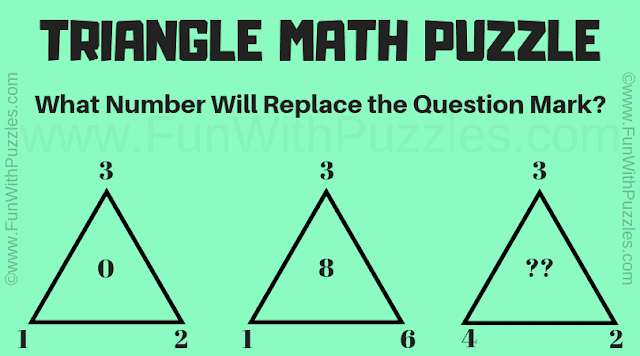## Wednesday, January 1, 2020

It is a very interesting triangle math puzzle to test your brainpower. Solving this triangle math puzzle will help you to improve your logical reasoning. It will also help you to improve your maths skills. In this triangle math puzzle, you are given some numbers around the triangle. Your challenge is to study the given number relationship and then find the value of the missing number which will replace the question mark.What number will replace the question mark?
The answer to this "Triangle Math Puzzle", can be viewed by clicking on the answer button.

1.You can also take the answer as 6 if you use the logic D=2(B+C-A)

1.Great. Thanks for coming up different answer and logic to this triangle maths puzzle.

2.Actually, your puzzle had taken by my daughter's teacher. She objected when we gave the answer 6 :)

3.These kinds of puzzles are open ended puzzles. It means that depending upon the correct logical reasoning, there could be more than one solutions to these puzzles. If someone thinks out of the box like you, one can come up with many different logical solutions to such #puzzles.

A comment doesn't cost a thing. Please drop a comment below to boost the author's morale.